## Direct torque control of Induction motor

##### Introduction

In this experiment, a sensorless speed control of an induction motor using direct torque control (DTC) will be simulated. The dq motor model developed in the previous experiment will be used for this as well. The estimator model and the switching algorithm will be modified to implement DTC. In addition to simulation, the controller will be evaluated on an actual induction motor in real-time.

##### Theoretical background

Motor model

The induction motor stator flux magnitude and position, electromagnetic torque, and speed is estimated from the stator voltages and currents as follows:

s,αβdt = Vs,αβ - RsIs,αβ(1)

θs = tan-1λs,βλs,α(2)

λs = √(λ2s,α + λ2s,β)(3)

Tem = 23×P2(Vs,αIs,β - Vs,βIs,α)(4)

λr,αβ = LrLms,αβ - σLsIs,αβ)(5)

θr = tan-1λr,βλr,α(6)

λr = √(λ2r,α + λ2r,β)(7)

ωr = rdt(8)

ωslip = 31×2P×Rr×Temλ2r(9)

ωmech = 2Pr - ωslip)(10)

where

1. Vs,αβ : stator voltage in αβ frame

2. Is,αβ : stator current in αβ frame

3. λs,αβ : estimated stator flux-linkage in αβ frame

4. λs : magnitude of estimated stator flux-linkage

5. θs : angle of estimated stator flux-linkage with respect to stator a-phase axis

6. λr,αβ : estimated rotor flux-linkage in αβ frame

7. λr : magnitude of estimated rotor flux-linkage

8. θr : angle of estimated rotor flux-linkage with respect to stator a-phase axis

9. Rs : stator resistance

10. Rr : reflected rotor resistance

11. Lm : Per-phase mutual inductance

12. Ls : Lls + Lm where, Lls is the stator leakage inductance

13. Lr : Llr + Lm where, Llr is the rotor leakage inductance

14. P : Number of stator poles

15. Tem : estimated output/electromagnetic torque

16. ωslip : estimated rotor electrical slip speed

17. ωr : estimated speed of rotor flux-linkage vector

18. ωmech : estimated rotor mechanical speed

19. σ : leakage factor given by σ = 1 - L2mLsLr

The αβ quantities are obtained from their corresponding abc by the following equations:

αβ = a + b × ej2π/3 + c × ej4π/3(11)

Once the mechanical speed, ωmech is estimated, the required electromagnetic torque needed to move the speed closer to desired speed can be obtained using a PI controller. The procedure to design the controller is same as done in earlier experiments. The output of the speed loop PI controller is the desired electromagnetic torque, Tem,ref. The system transfer function is as shown: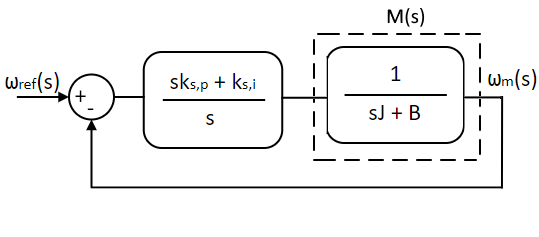The switching states of the inverter for DTC is determined based on the following three variables:

1. error in the desired and estimated electromagnetic torque, δTem = Tem,ref - Tem

2. error in the desired and estimated stator flux-linkage magnitude, δλs = λs,ref - λs

3. current position of the stator flux-linkage vector, θs

The principle is that, if the error in the torque is positive and error in flux-linkage is negative, the switching state is chosen such that the electromagnetic torque increases while the stator flux-linkage decreases. Consider for instance θs is between -π/3 and +π/3. For this condition, the effect of each inverter switching state on the electromagnetic torque and the stator flux-linkage is given in the table:

Switch state Tem λs
A B C
ON OFF OFF Indeterminate Indeterminate
ON ON OFF Increase Increase
OFF ON OFF Increase Decrease
OFF ON ON Indeterminate Indeterminate
OFF OFF ON Decrease Decrease
ON OFF ON Decrease Increase
OFF OFF OFF Decrease/Increase No change
OFF OFF OFF Decrease/Increase No change

Similarly, the effect of each inverter switching state on the Tem and the λs can be determined based for all values of θs as illustrated in reference 1. Applying zero vector in the above case, leads to decrease in Tem if speed is positive (counter-clockwise) and increase in Tem if speed is negative. Of the eight switching combination, only switching states which produce determinate effect is chosen to regulate motor torque and flux.

##### Simulation of Induction motor speed control

The above speed control model is simulated using Workbench in this section.

1. Open Workbench and pin the Explorer and Properties dock.

2. Navigate to and open the IMDTCControl project file in Experiment5\Simulation folder usually in the following location: C:\Program Files (x86)\Sciamble\WorkBench v1\Examples\CUSPLab\AdvancedDrives.

3. The Script file computes the reference stator flux-linkage at rated operating confition using Eqns. 1 through 10. In addition, the speed PI controller gains are computed as well for a phase margin of 60° and cross-over frequency of 25 rad/s. Change these parameters as desired to test the motor under different conditions.

4. Open the model file: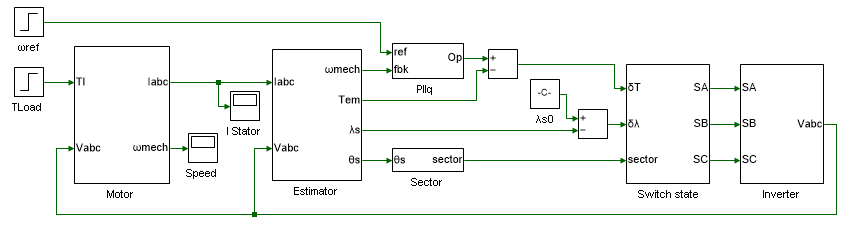The left-most section contains the induction motor dq model designed in the previous experiment. The electromagnetic torque, rotor mechanical speed and stator flux linkage are estimated in the Estimator subsystem using Eqns. 1 through 10. The desired torque is obtained from the estimated speed and the desired reference speed using a PI controller, the output of which is the desired electromagnetic torque. The switching states of the inverter is determined in the Switch state subsystem based on the error in the desired and estimated electromagnetic torque and stator flux-linkage. Finally, these switching states are applied to the mathematical model of an ideal 3Φ inverter in Inverter subsystem to obtain the induction motor applied terminal voltages.

5. Run the simulation model and observe the stator current, speed and output torque.

6. Modify the PI controller gain values to generate underdamped and overdamped response. Rerun the simulation and verify the results.

7. Adjust the torque and flux hysteresis band within the Switch state subsystem to the values listed below and rerun the simulation.

Tool name Property field Property value Comments
Hysteresis Lower threshold -IMParam:δλ * 2 Stator flux linkage hysteresis band.
Hysteresis Upper threshold IMParam:δλ * 2 Stator flux linkage hysteresis band.
Hysteresis 1 Lower threshold -IMParam:δTem * 2 Electromagnetic torque hysteresis band.
Hysteresis 1 Upper threshold IMParam:δTem * 2 Electromagnetic torque linkage hysteresis band.

8. Observe the current and speed results. Repeat the previous step while gradually increasing the band, until the system becomes unstable.

This concludes the simulation of induction motor direct torque control. In the following section, a pre-built induction motor direct torque control model is run in real-time.

##### Real-time vector control of Induction motor
1. Open Workbench and pin the Explorer and Properties dock.

2. Navigate to and open the IMDTCControl project file in Experiment5\Realtime folder usually in the following location: C:\Program Files (x86)\Sciamble\WorkBench v1\Examples\CUSPLab\AdvancedDrives.

3. Expand the project in the Explorer and open the ModelFile model file shown below: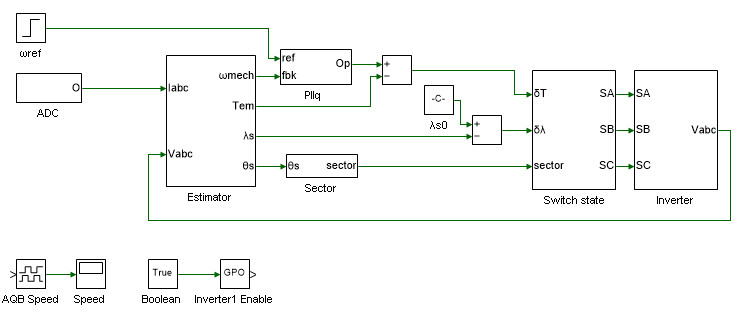The model is same as the one used in simulation expect for the induction motor dq model which has been replaced by feedback stator currents and motor speed measured from an actual induction motor to be controlled in real-time.

4. The Init() function within the IMParam script file computes the controller parameters based on the motor parameters, and the loop cross-over frequency and phase margin. Set the speed loop cross-over frequency ωc and the phase margin φm in the script to the same value used in simulation.

5. The Init() function must be called prior to running the simulation model to initialize the controller gain values. To do this open project properties, double click on the IMabc project node in the Explorer, and set the Prerun Model/Function to IMParam:Init().

Similarly set the Start model/Function to ModelFile, to run the model after parameter initialization.

6. Click the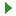button to run the model in simulation mode. Using the DataPeek, verify that the controller gain parameters computed by the Init() function matches that of values computed earlier.

7. Turn ON the DC power supply and set the voltage to 40 V.

8. Ensure that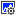button on the top dock, to transition to real-time mode, is pressed. Click the run button.

9. Observe the speed and current result in the scope. Click the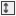to focus the result. After about 5 s, stop data logging by clicking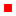.

10. Turn OFF the DC power supply.

11. If necessary, repeat the experiment for different loop cross-over frequencies and phase margins.

12. Turn OFF the DC power supply and disconnect all the connections including the USB.

##### Reference
1. "Advanced Electric Drives: Analysis, Control, and Modeling Using MATLAB/Simulink," Ned Mohan, Wiley Publication.

##### Lab report and reading assignment
1. List the kp and ki values of the speed PI controller.

2. Attach the plot of 3φ stator and motor speed from simulation.

3. The model does not employ zero switching states. Modify the simulation to include these states as well.

4. List all the switching states for all values of stator flux-linkage position and list their effect on the electromagnetic torque and the stator flux linkage magnitude.

5. Attach the motor speed from real-time.

6. If there are any performance difference between vector control (previous experiment) and DTC, explain the cause.